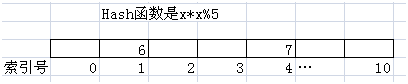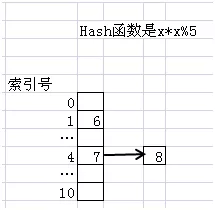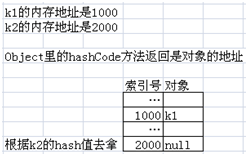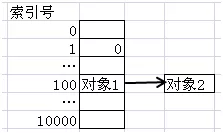#### 1. 通过Hash算法来了解HashMap对象的高效性2. 为什么要重写equals和hashCode方法

import java.util.HashMap;

class Key {
private Integer id;

public Key(Integer id) {
this.id = id;
}

public Integer getId() {
return id;
}

//故意先注释掉equals和hashCode方法
// public boolean equals(Object o) {
// if (o == null || !(o instanceof Key))
// { return false; }
// else
// { return this.getId().equals(((Key) o).getId());}
// }

// public int hashCode()
// { return id.hashCode(); }
}

public class WithoutHashCode {
public static void main(String args) {
Key k1 = new Key(1);
Key k2 = new Key(1);
HashMap<Key, String> hm = new HashMap<Key, String>();
hm.put(k1, "Key with id is 1");
System.out.println(hm.get(k2));
}
}HashMap是用链地址法来处理冲突，也就是说，在100号位置上，有可能存在着多个用链表形式存储的对象。它们通过hashCode方法返回的hash值都是100。